SummaryCustom SearchSUMMARY The important points of this chapter are summarized below. Study this information before continuing, as this information will lay the foundation for later chapters. INDUCTANCE - The characteristic of an electrical circuit that opposes a change in current. The reaction (opposition) is caused by the creation or destruction of a magnetic field. When current starts to flow, magnetic lines of force are created. These lines of force cut the conductor inducing a counter emf in a direction that opposes current.SELF-INDUCTANCE - The process by which a circuit induces an emf into itself by its own moving magnetic field. All electrical circuits possess self-inductance. This opposition (inductance), however, only takes place when there is a change in current. Inductance does NOT oppose current, only a CHANGE in current. The property of inductance can be increased by forming the conductor into a loop. In a loop, the magnetic lines of force affect more of the conductor at one time. This increases the self-induced emf.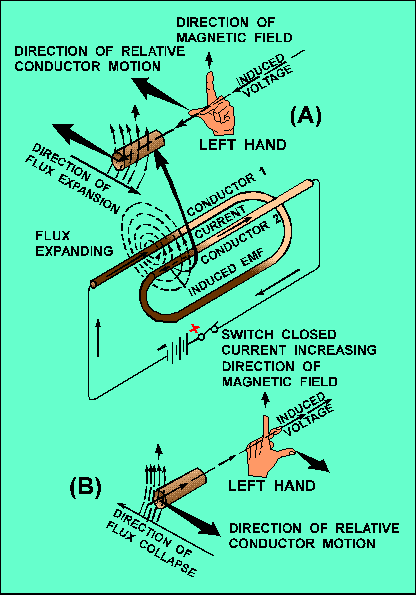INDUCTANCE OF A COIL - The property of inductance can be further increased if the conductor is formed into a coil. Because a coil contains more loops, more of the conductor can be affected by the magnetic field. Inductors (coils) are classified according to core type. The core material is normally either air or soft iron.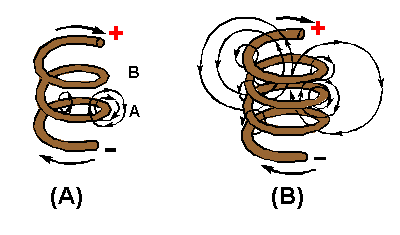FACTORS AFFECTING COIL INDUCTANCE - The inductance of a coil is entirely dependent upon its physical construction. Some of the factors affecting the inductance are: The number of turns in the coil. Increasing the number of turns will increase the inductance.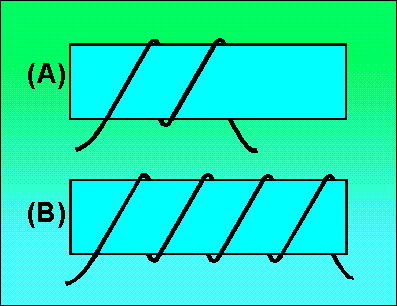The coil diameter. The inductance increases directly as the cross-sectional area of the coil increases.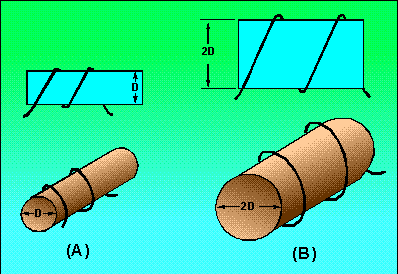The length of the coil. When the length of the coil is increased while keeping the number of turns the same, the turn-spacing is increased. This decreases the inductance of the coil.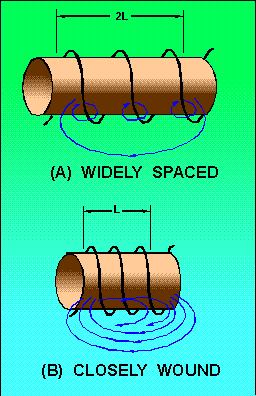The type of core material. Increasing the permeability of the core results in increasing the inductance of the coil.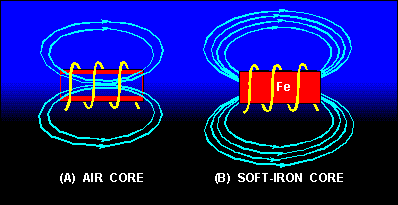Winding the coil in layers. The more layers used to form a coil, the greater effect the magnetic field has on the conductor. By layering a coil, you can increase the inductance.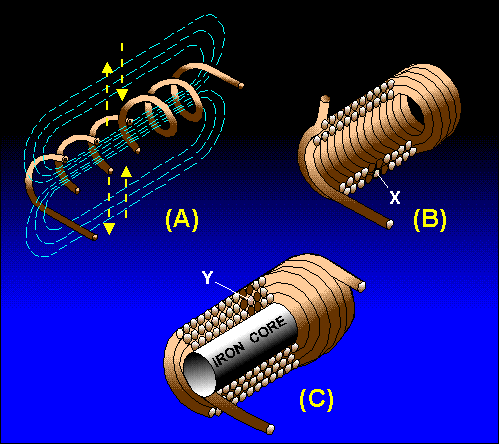UNIT OF INDUCTANCE - Inductance (L) is measured in henrys (H). An inductor has an inductance of one henry (H) if an emf of one volt is induced in the inductor when the current through the inductor is changing at the rate of 1 ampere per second. Common units of inductance are henry (H), millihenry (mH) and the microhenry (μH). GROWTH AND DECAY OF CURRENT IN AN LR CIRCUIT - The required for the current in an inductor to increase to 63.2 percent of the maximum current or to decrease to 36.8 percent of the maximum current is known as the time constant. The letter symbol for an LR time constant is L/R. As a formula: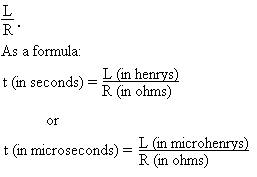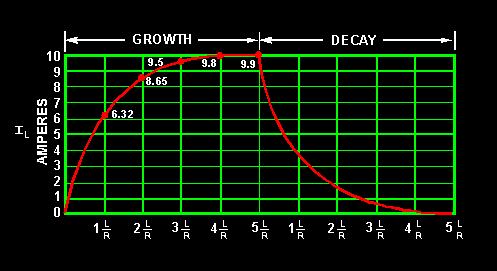The time constant of an LR circuit may also be defined as the time required for the current in the inductor to grow or decay to its final value if it continued to grow or decay at its initial rate. For all practical purposes, the current in the inductor reaches a maximum value in 5 "Time Constants" and decreases to zero in 5 "Time Constants". POWER LOSSES IN AN INDUCTOR - Since an inductor (coil) contains a number turns of wire, and all wire has some resistance, the inductor has a certain amount of resistance. This resistance is usually very small and has a negligible effect on current. However, there are power losses in an inductor. The main power losses in an inductor are copper loss, hysteresis loss, and eddy-current loss. Copper loss can be calculated by multiplying the square of the current by the resistance of the wire in the coil (I2R). Hysteresis loss is due to power that is consumed in reversing the magnetic field of the core each time the current direction changes. Eddy-current loss is due to core heating caused by circulating currents induced in an iron core by the magnetic field of the coil. MUTUAL INDUCTANCE - When two coils are located so that the flux from one coil cuts the turns of the other coil, the coils have mutual inductance. The amount of mutual inductance depends upon several factors: the relative position of the axes of the two coils; the permeability of the cores; the physical dimensions of the two coils; the number of turns in each coil, and the distance between the coils. The coefficient of coupling K specifies the amount of coupling between the coils. If all of the flux from one coil cuts all of the turns of the other coil, the coefficient of coupling K is 1 or unity. If none of the flux from one coil cuts the turns of the other coil, the coefficient of coupling is zero. The mutual inductance between two coils (L1 and L2) may be expressed mathematically as: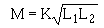COMPUTING THE INDUCTANCE OF A CIRCUIT - When the total inductance of a circuit is computed, the individual inductive values are treated the same as resistance values. The inductances of inductors in series are added like the resistances of resistors in series. That is,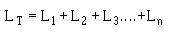The inductances of inductors in parallel are combined mathematically like the resistances of resistors in parallel. That is,Both of the above formulas are accurate, providing there is no mutual inductance between the inductors.

Privacy Statement - Copyright Information. - Contact UsIntegrated Publishing, Inc.